## 走进哈希心房

article2023/12/11 13:07:23/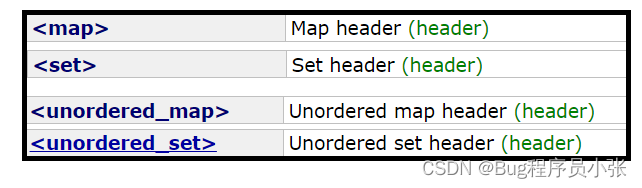# 哈希的概念

map和set的查找效率对于我们而言已经是很满足了，但是依然达不到大佬们的标准，于是它们想构造一种不需要比较，一次直接从表中获取元素的搜索方法。

# 哈希函数

●哈希函数的定义域必须包括需要存储的全部关键码，如果哈希表中允许有n个地址时，其值域必须在0到n-1之间。

●哈希函数计算出来的地址需要的均匀的分布在整个空间中。

●直接定址法

●除留余数法

# 哈希冲突和解决方法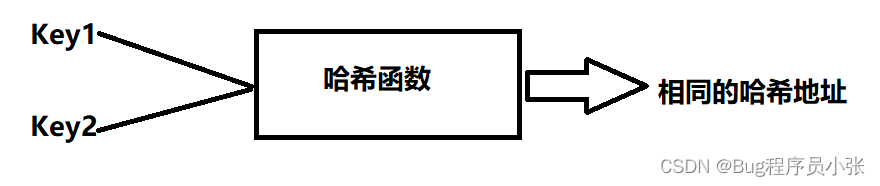## 闭散列

●将近似整型的类型强转成无符号整数。

``````template <class K>
struct HashFun
{
size_t operator()(const K& key)
{
return (size_t)key;
}
};``````

●特化字符串类型，将字符串中每个字符的ASCII值加在一起。*=31是为了减少冲突。

``````template <>
struct HashFun<string>
{
size_t operator()(const string& str)
{
size_t  num = 0;
for (auto ch : str)
{
ch *= 31;
num += ch;
}

return num;
}
};``````

``````    enum State
{
EMPTY,
EXIST,
DELETE,
};``````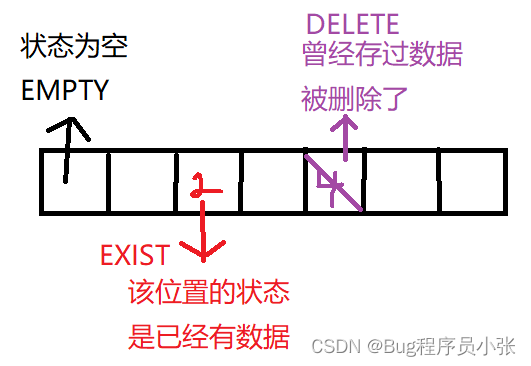节点结构：要存储的数据和存储位置状态的标记，标记初始状态为空。

``````	template <class K,class V>
struct HashDate
{
//存储数据
pair<K, V> _kv;
//表状态
State _state = EMPTY;
};``````

HashTable结构：存储HashDate的容器和一个记录容器中存储有效数据的个数。

``````	template<class K,class V,class Hash = HashFunc<K>>
class HashTable
{
typedef HashDate<K, V> Data;
public:
HashTable()
:_n(0)
{
//有效数据个数初始为10
_table.resize(10);
}
private:
vector<Data> _table;
size_t _n = 0;
};``````

### 插入

●判断该数据是否已经存在于表中，以存在直接返回false。（复用了查找接口）

●新数据不在表中，插入前判断是否需要扩容：

1.当负载因子超标进行一次扩容，负载因子的计算公式：表中元素个数/总空间，当元素个数已经是总容量的70%甚至更多时，进行扩容。

2.按照习惯，通常会将容量扩至原来的二倍，那么扩容后又带来了新的问题，每个数据在新表中的映射位置可能和旧表不同。

3.处理方法，创建一个新的HashTable,将旧表中的数据全部插入到新建的HashTable中，最后来一个“偷梁换柱”，交换新旧表。

4.这里需要说明一下，HashTable的结构中有一个存储数据的vector和一个记录有效数据个数的变量，在局部对象生命周期结束调用其析构时，默认生成的析构就能够完成任务，因为vector会调用其自己的析构函数，内置类型_n销毁时不需要资源清理，最后系统直接将其内存回收即可

●插入部分，这里采用的插入方法是线性探测，如果映射位置为空或者删除状态直接进行插入，如果映射位置被占用，向后继续查找直至找到空的位置，如果查找到末尾还未找到合适位置，此时注意处理Hash_i从头进行查找。

``````		//插入数据
bool insert(const pair<K,V>& kv)
{
if (Find(kv.first))
{
return false;
}
//负载因子超标，需要扩容,元素个数/表总空间的个数
if (_n * 10 / _table.size() >= 7)
{
cout << "扩容成功" << endl;
//需要扩容
HashTable<K, V, Hash> newHT;
newHT._table.resize(_table.size() * 2);
//扩容后存在，数据的位置可能改变
for (auto& e : _table)
{
if (e._state == EXIST)
{
newHT.insert(e._kv);
}
}
//交换新旧表
_table.swap(newHT._table);
}
Hash sh;
size_t Hash_i = sh(kv.first) % _table.size();
//size_t Hash_i = kv.first % _table.size();
//如果映射位置的状态不是占用
while(_table[Hash_i]._state == EXIST)
{
//向后找空位置
++Hash_i;
//防止越界
Hash_i = Hash_i % _table.size();
}

//走到这里该位置为空或者被占用过，插入
_table[Hash_i]._kv = kv;
_table[Hash_i]._state = EXIST;

++_n;
return true;
}``````

### 查找

●根据Key值在_table中的映射直接查找。

●如果映射位置为空，说明表中没要要查找的值。

●映射位置被占且该位置的值不是我们要查找的，或者是删除状态，那么从此位置开始向后开始查找，就可以找到我们要查找的值了。

``````		//查找
Data* Find(const K& key)
{
Hash sh;
size_t Hash_i = sh(key) % _table.size();
while (_table[Hash_i]._state != EMPTY)
{
if (_table[Hash_i]._state == EXIST &&
_table[Hash_i]._kv.first == key)
{
return &_table[Hash_i];
}

++Hash_i;
Hash_i %= _table.size();
}

return nullptr;
}``````

### 删除

●调用查找接口，判断要删除的数据表中是否存在。

●删除目标存在表中，进行删除：注意这里要做的处理并不是真的删除，而是改变该位置的状态为DELETE,这样一来如果有新的数据插就直接覆盖在这里。

``````		//删除
bool earse(const K& key)
{
Data* ret = Find(key);
if (ret == nullptr)
{
return false;
}

ret->_state = DELETE;
_n--;
cout << "删除成功" << endl;
return true;
}``````

## 开散列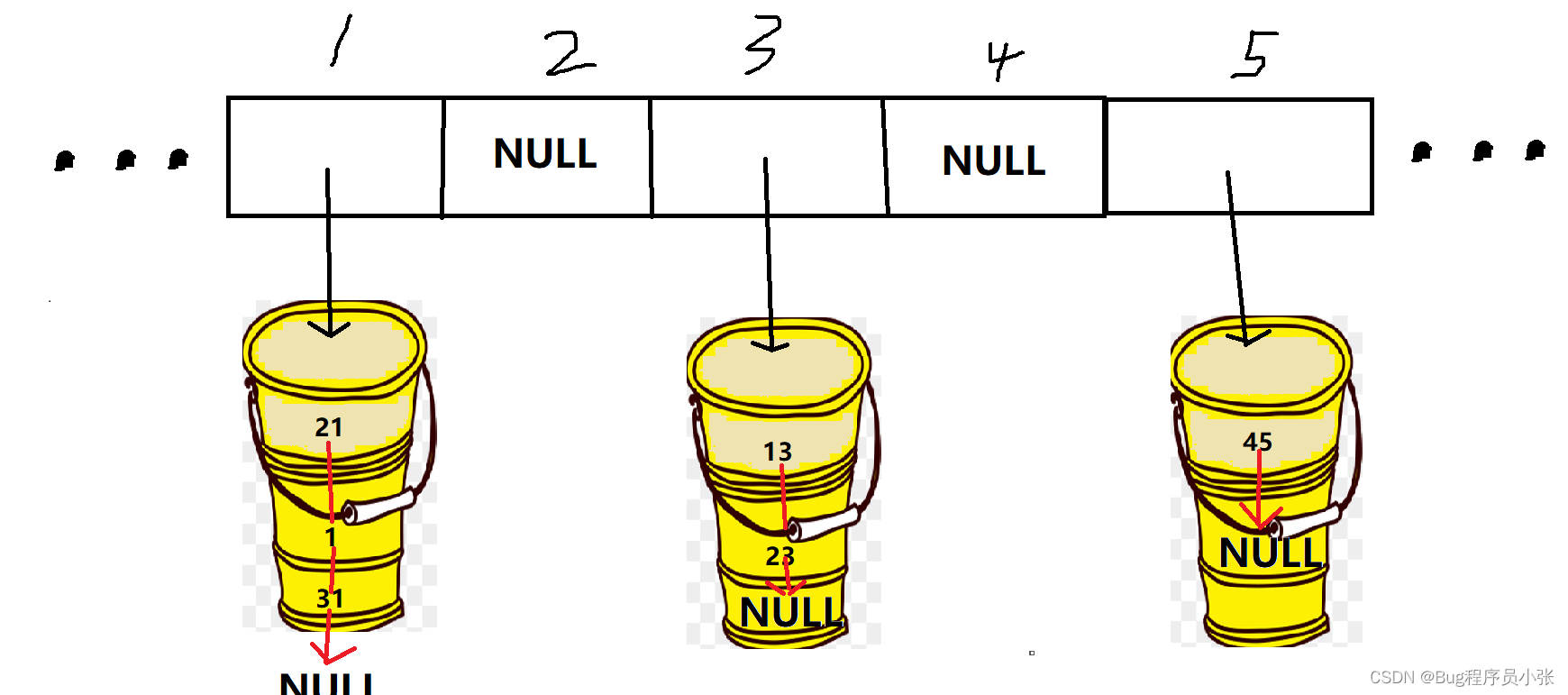### 插入

●第一步检查要插入数据是否已经在存在于表中。

●开散列的逻辑结构通过上图你一定有了一些了解，这里的扩容条件是通过对有效元素个数和容量的比较，当二者相等时进行一次扩容：

1.首先需要注意的是，哈希桶结构中表里存的是单链表的头节点，每个单链表可能有多个数据，这时的析构函数就需要显示定义，代码实现参考文章最后的整体实现！

2.按照闭散列的思路去获取旧节点的值，在申请节点插入到新表中也是可以的，但是考虑到旧表的节点没用直接销毁了，新表又需要新的节点，索性将旧表的节点拆下来拿给新表用。

3.节点插入时使用的方法是头插，新节点作为一个“桶”的头节点存储在哈希表中。

``````        bool Insert(const pair<K, V>& kv)
{
if (Find(kv.first))
{
return false;
}
//扩容的情况
if (_n == _tables.size())
{
vector<Node*> newTB;
newTB.resize(_tables.size() * 2, nullptr);

for (size_t i = 0; i < _tables.size(); i++)
{
Node* cur = _tables[i];
while (cur)
{
Node* Next = cur->_next;
size_t Hashi = Hash()(cur->_kv.first) % newTB.size();

cur->_next = newTB[Hashi];
newTB[Hashi] = cur;

cur = Next;
}
_tables[i] = nullptr;
}
}
//节点插入，头插，挂节点
size_t hashi = Hash()(kv.first) % _tables.size();

Node* newNode = new Node(kv);
newNode->_next = _tables[hashi];
_tables[hashi] = newNode;

++_n;

return true;
}``````

### 查找

``````		Node* Find(const K& key)
{
size_t hashi = Hash()(key) % _tables.size();
Node* cur = _tables[hashi];
while (cur)
{
if (cur->_kv.first == key)
{
return cur;
}
cur = cur->_next;
}

return nullptr;
}``````

### 删除

1.首先判断映射位置有没有桶。

2.找到对应的桶后，如果该值在头部，直接删除即可。

3.要删除的值在链表中间，需要一个记录节点前一节点的指针prev,找到要删除的节点后，prev->next指向cur->next;

``````		bool Earse(const K& key)
{
size_t hashi = Hash()(key.first) % _tables.size();
Node* prev = nullptr;
Node* cur = _tables[hashi];

while (cur)
{
if (cur->_kv.first == key)
{
//删除
if (cur == _tables[hashi])
{
_tables[hashi] = cur->_next;
}
else
{
Node* Next = cur->_next;
prev->_next = Next;
}
--_n;
delete cur;
return true;
}
else
{
prev = cur;
cur = cur->_next;
}
}
return false;
}``````

### 哈希表（开散列）整体代码

``````template <class K>
struct HashFun
{
size_t operator()(const K& key)
{
return (size_t)key;
}
};
template <>
struct HashFun<string>
{
size_t operator()(const string& str)
{
size_t  num = 0;
for (auto ch : str)
{
ch *= 31;
num += ch;
}

return num;
}
};

template <class K, class V>
struct HashBucketNode
{
pair<K, V> _kv;
HashBucketNode<K, V>* _next;

HashBucketNode(const pair<K, V>& kv)
:_kv(kv)
, _next(nullptr)
{}
};

template <class K, class V, class Hash = HashFun<K>>
class HashBucket
{
typedef HashBucketNode<K, V> Node;
public:
HashBucket()
:_n(0)
{
_tables.resize(10);
}
~HashBucket()
{
for (size_t i = 0; i < _tables.size(); ++i)
{
Node* cur = _tables[i];
while (cur)
{
Node* Next = cur->_next;

delete cur;
cur = Next;
}
_tables[i] = nullptr;
}
cout << "调用析构函数" << endl;
}
bool Insert(const pair<K, V>& kv)
{
if (Find(kv.first))
{
return false;
}
if (_n == _tables.size())
{
vector<Node*> newTB;
newTB.resize(_tables.size() * 2, nullptr);

for (size_t i = 0; i < _tables.size(); i++)
{
Node* cur = _tables[i];
while (cur)
{
Node* Next = cur->_next;
size_t Hashi = Hash()(cur->_kv.first) % newTB.size();

cur->_next = newTB[Hashi];
newTB[Hashi] = cur;

cur = Next;
}
_tables[i] = nullptr;
}
}
//节点插入，头插，挂节点
size_t hashi = Hash()(kv.first) % _tables.size();

Node* newNode = new Node(kv);

newNode->_next = _tables[hashi];
_tables[hashi] = newNode;

++_n;

return true;
}

Node* Find(const K& key)
{
size_t hashi = Hash()(key) % _tables.size();
Node* cur = _tables[hashi];
while (cur)
{
if (cur->_kv.first == key)
{
return cur;
}
cur = cur->_next;
}

return nullptr;
}
bool Earse(const K& key)
{
size_t hashi = Hash()(key.first) % _tables.size();
Node* prev = nullptr;
Node* cur = _tables[hashi];

while (cur)
{
if (cur->_kv.first == key)
{
//删除
if (cur == _tables[hashi])
{
_tables[hashi] = cur->_next;
}
else
{
Node* Next = cur->_next;
prev->_next = Next;
}
--_n;
delete cur;
return true;
}
else
{
prev = cur;
cur = cur->_next;
}
}
return false;
}
private:
//指针数组
vector<Node*> _tables;
size_t _n = 0;
};``````

# 位图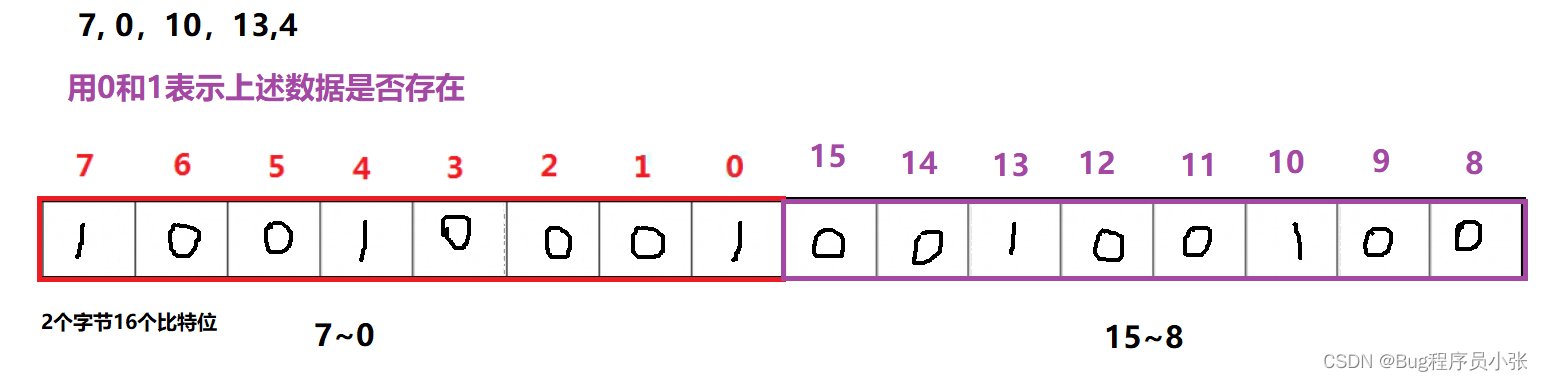## 位图模拟实现思路分析：

●N表示要处理数据的最大范围，成员方面用一个存储char的容器模拟实现。容器初始大小设置为N/8+1,意义是要处理的数据范围需要多少个char才能够每个数据都有与之对应的映射(用一个比特位来表示一个数据是否存在)。N/8可能会有余数，多开一个char保证空间够用。

``````template<size_t N>
class BitSet
{
public:
BitSet()
{
_bitset.resize(N/8+1,0);
}
private:
vector<char> _bitset;
};``````

●当新数据进入到位图中，i记录该数据x在容器中的哪一个char中，j计算x在这个char中的哪一个比特位。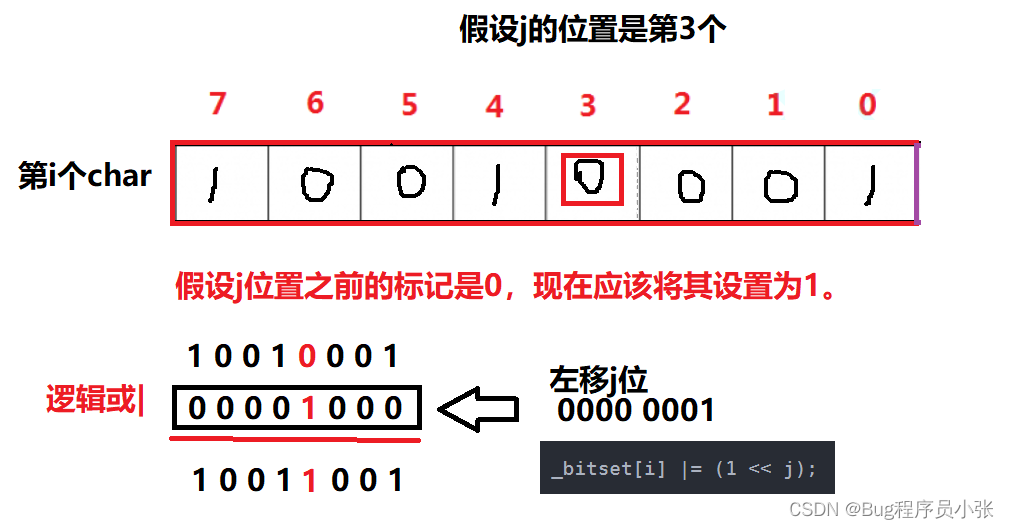``````    void insert(size_t x)
{
size_t i = x / 8;
size_t j = x % 8;

_bitset[i] |= (1 << j);
}``````

●当要将数据x在位图中去掉时，同样的要知道其在哪一个char中，在该char中的比特位的位置。如果该位置是1将其改为0。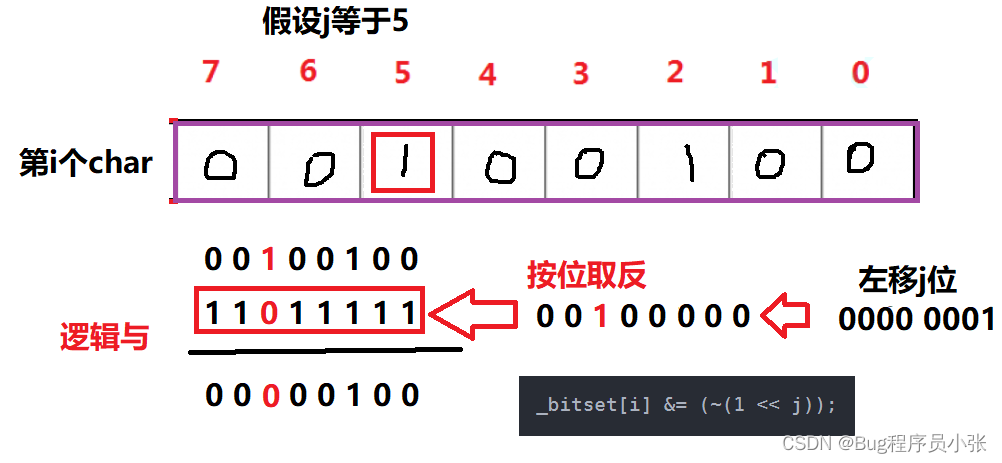``````	void earse(size_t x)
{
size_t i = x / 8;
size_t j = x % 8;

_bitset[i] &= (~(1 << j));
}``````

●查找一个数据是否在位图中有存在标记，首先还是要记录i,j两个位置和上述意义相同。这里的返回值是一个布尔值，如果不存在会返回0,存在会返回一个非0的数据。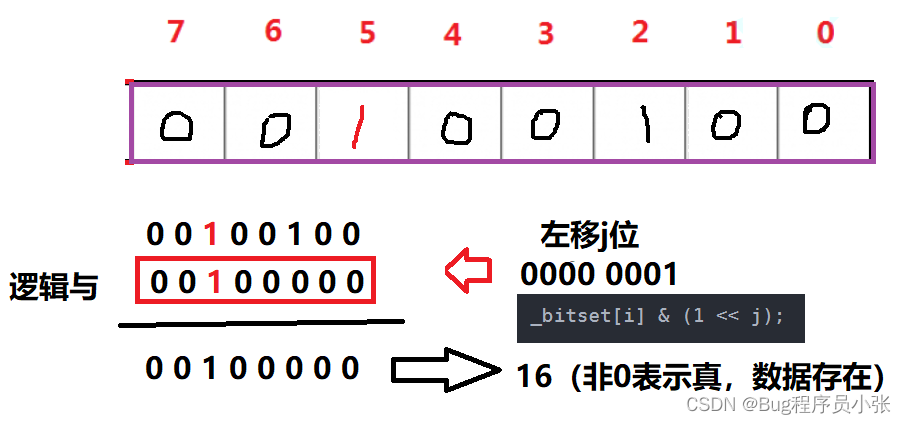``````	bool Find(size_t x)
{
size_t i = x / 8;
size_t j = x % 8;

return _bitset[i] & (1 << j);
}``````

``````​template<size_t N>
class BitSet
{
public:
BitSet()
{
_bitset.resize(N/8+1,0);
}
void insert(size_t x)
{
size_t i = x / 8;
size_t j = x % 8;

_bitset[i] |= (1 << j);
}
void earse(size_t x)
{
size_t i = x / 8;
size_t j = x % 8;

_bitset[i] &= (~(1 << j));
}
bool Find(size_t x)
{
size_t i = x / 8;
size_t j = x % 8;

return _bitset[i] & (1 << j);
}
private:
vector<char> _bitset;
};
``````

## 位图应用

512M左右就可以表示数据是否存在了。

00表示两文件中都没有某个数据，10表示在一个位图中出现过，01表示在两个位图中都出现了，这种状态也就是交集数据的状态。

``````template<size_t N>
class twobitset
{
public:
void Insert(size_t x)
{
if (!_bit1.Find(x) && !_bit2.Find(x))//00
{
_bit2.insert(x);
}
else if (!_bit1.Find(x) && _bit2.Find(x))//01 出现了一次
{
_bit1.insert(x);
_bit2.earse(x);//10 表示出现2次及以上，两个表中都有
}
}
void _Print_one()
{
//打印值出现一次的
for (size_t i = 0; i < N; i++)
{
if (!_bit1.Find(i) && _bit2.Find(i))//01
{
cout << i << endl;
}
}
cout << endl;
}
void _Print_more()
{
//出现两次或以上
for (size_t i = 0; i < N; i++)
{
if (_bit1.Find(i) && !_bit2.Find(i))//10
{
cout << i << endl;
}
}
cout << endl;
}
private:
BitSet<N> _bit1;
BitSet<N> _bit2;
};

void Testtwobit()
{
twobitset<2000> tb;
int a[] = { 520,43,132,454,43,43,1314,88,88,132};
for (auto e : a)
{
tb.Insert(e);
}
cout << "出现一次的数据有:>"<<endl;
tb._Print_one();

cout << "出现2次及以上的数据有:>"<<endl;
tb._Print_more();
}``````

# 布隆过滤器

●不同的哈希函数返回不同的映射位置：

``````//在Brian Kernighan与Dennis Ritchie的《The C Programming Language》一书被展示而得名
struct BKDRHash
{
size_t operator()(const string& str)
{
size_t num = 0;
for (auto ch : str)
{
ch *= 31;
num += ch;
}

return num;
}
};

//Arash Partow发明的一种hash算法。
struct APHash
{
size_t operator()(const string& key)
{
size_t hash = 0;
int i = 0;

for (auto ch : key)
{
if ((i & 1) == 0)
{
hash ^= ((hash << 7) ^ (ch) ^ (hash >> 3));
}
else
{
hash ^= (~((hash << 11) ^ (ch) ^ (hash >> 5)));
}

++i;
}

return hash;
}
};
//Daniel J.Bernstein教授发明的一种hash算法。
struct DJBHash
{
size_t operator()(const string& key)
{
size_t hash = 5381;

for (auto ch : key)
{
hash += (hash << 5) + ch;
}

return hash;
}
};
// 由Justin Sobel发明的一种hash算法。
struct JSHash
{
size_t operator()(const string& s)
{
size_t hash = 1315423911;
for (auto ch : s)
{
hash ^= ((hash << 5) + ch + (hash >> 2));
}
return hash;
}
};``````

N代表数据的最大范围，X表示存储一个数据需要的比特位数，K表示key的数据类型，不同的哈希函数返回不同的。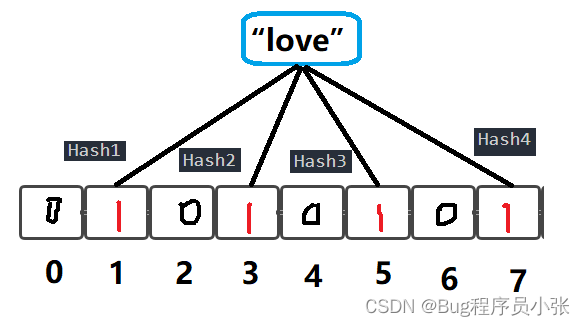如上图所示，通过不同的哈希函数将“love”数据映射到该图中，但是这也埋下了布隆过滤器不能100%确定一个数据存在的原因（及时test返回的结果是肯定的）。看下图：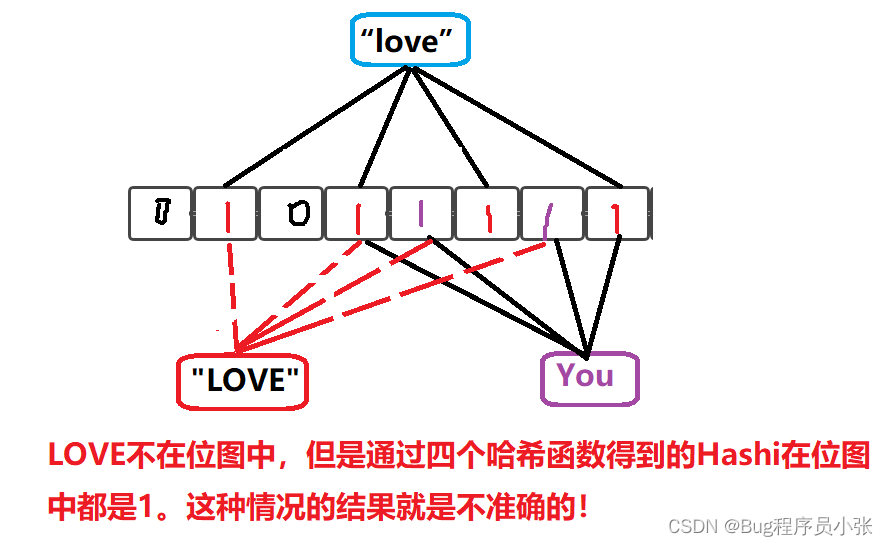小总结：布隆过滤器告诉我们一个值不在，就是真的不在。如果它告诉我们一个数据存在，只能代表存在的可能性很大！

``````template <size_t N, size_t X = 5, class K = string,
class HashFun1 = BKDRHash,
class HashFun2 = APHash,
class HashFun3 = DJBHash,
class HashFun4 = JSHash
>
class Bloom_Filter
{
void set(const K& key)
{
size_t Hash1 = BKDRHash()(key) % (N * X);
size_t Hash2 = APHash()(key) % (N * X);
size_t Hash3 = DJBHash()(key) % (N * X);
size_t Hash4 = JSHash()(key) % (N * X);

_bit.set(Hash1);
_bit.set(Hash2);
_bit.set(Hash3);
_bit.set(Hash4);
}
bool test(const K& key)
{
size_t Hash1 = BKDRHash()(key) % (N * X);
size_t Hash2 = APHash()(key) % (N * X);
size_t Hash3 = DJBHash()(key) % (N * X);
size_t Hash4 = JSHash()(key) % (N * X);
//Hash1位置不存在
if (!_bit.test(Hash1))
{
return false;
}
if (!_bit.test(Hash2))
{
return false;
}
if (!_bit.test(Hash3))
{
return false;
}
if (!_bit.test(Hash4))
{
return false;
}
//上述返回不存在的结果是准确的

//四个映射位置都存在，返回true，这个true是不准确的
//可能一个数据的四个映射位置都与其它元素冲突
return true;
}
private:
std::bitset<N* X> _bit;
};``````

``````void test_bloomfilter1()
{
string str[] = { "特战尖刀", "李云龙", "楚云飞", "张大彪", "营长1","营长2","1营长","营11长","1营长1" };
Bloom_Filter<10> bf;
for (auto& str : str)
{
bf.set(str);
}

//查找的数据都在位图中
cout << "查找在位图中的数据:>"<<endl;
for (auto& s : str)
{
cout << bf.test(s) << " ";
}
cout << endl;

//查找的数据不在位图中
cout << "查找不在位图中的数据:>"<<endl;
srand(time(0));
for (const auto& s : str)
{
cout << bf.test(s + to_string(rand())) << " ";
}
}``````### K_A18_001 基于STM32等单片机采集MQ2传感参数串口与OLED0.96双显示

K_A18_001 基于STM32等单片机采集MQ2传感参数串口与OLED0.96双显示一、资源说明二、基本参数参数引脚说明三、驱动说明IIC地址/采集通道选择/时序对应程序:四、部分代码说明1、接线引脚定义1.1、STC89C52RCMQ2传感参模块1.2、STM32F103C8T6MQ2传感参模块五、基础知识学习与相关…

### 让你少写多做的 ES6 技巧

Array.of 关于奇怪的 Array 函数&#xff1a; 众所周知&#xff0c;我们可以通过Array函数来做以下事情。 初始化一个指定长度的数组。 设置数组的初始值。 // 1. Initialize an array of the specified length const array1 Array(3) // [ , , ] // 2. Set the initial value…

### SQLMap 源码阅读

0x01 前言 还是代码功底太差&#xff0c;所以想尝试阅读 sqlmap 源码一下&#xff0c;并且自己用 golang 重构&#xff0c;到后面会进行 ysoserial 的改写&#xff1b;以及 xray 的重构&#xff0c;当然那个应该会很多参考 cel-go 项目 0x02 环境准备 sqlmap 的项目地址&…